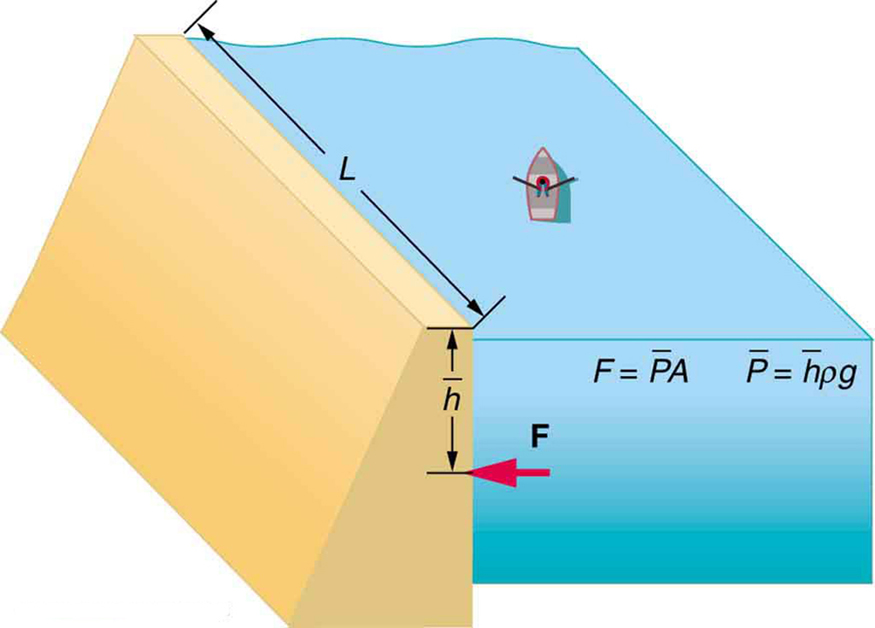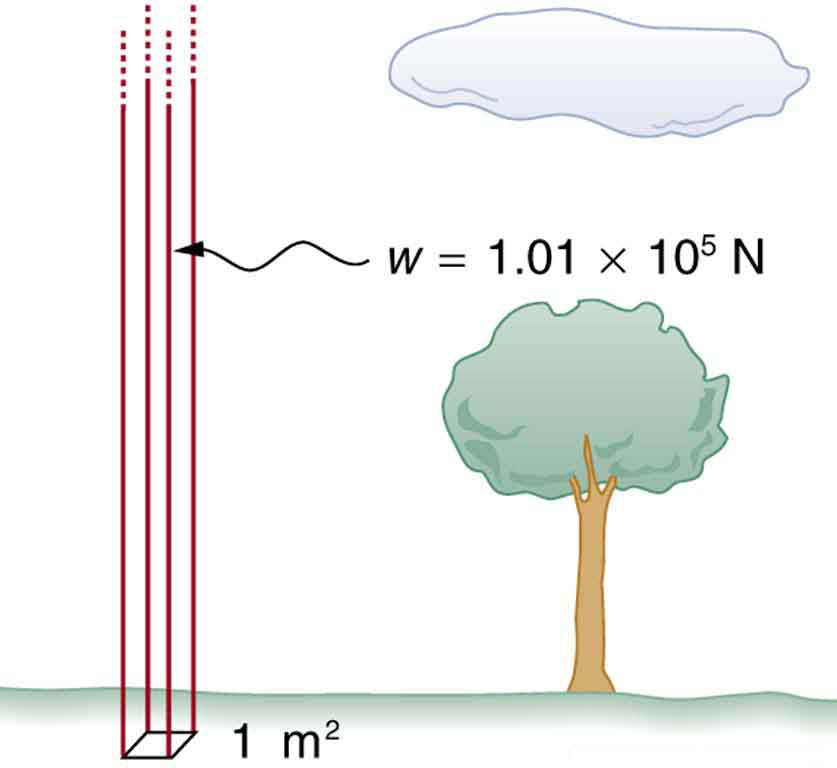# 11.4 Variation of pressure with depth in a fluid  (Page 2/4)

 Page 2 / 4The dam must withstand the force exerted against it by the water it retains. This force is small compared with the weight of the water behind the dam.

Atmospheric pressure is another example of pressure due to the weight of a fluid, in this case due to the weight of air above a given height. The atmospheric pressure at the Earth’s surface varies a little due to the large-scale flow of the atmosphere induced by the Earth’s rotation (this creates weather “highs” and “lows”). However, the average pressure at sea level is given by the standard atmospheric pressure ${P}_{\text{atm}}$ , measured to be

$\text{1 atmosphere (atm)}={P}_{\text{atm}}=1.01×{\text{10}}^{5}\phantom{\rule{0.25em}{0ex}}{\text{N/m}}^{2}=\text{101 kPa}.$

This relationship means that, on average, at sea level, a column of air above $1.00\phantom{\rule{0.25em}{0ex}}{\text{m}}^{2}$ of the Earth’s surface has a weight of $1.01×{\text{10}}^{5}\phantom{\rule{0.25em}{0ex}}\text{N}$ , equivalent to $\text{1 atm}$ . (See [link] .)Atmospheric pressure at sea level averages 1 . 01 × 10 5 Pa size 12{1 "." "01" times "10" rSup { size 8{5} } "Pa"} {} (equivalent to 1 atm), since the column of air over this 1 m 2 size 12{1m rSup { size 8{2} } } {} , extending to the top of the atmosphere, weighs 1 . 01 × 10 5 N size 12{1 "." "01" times "10" rSup { size 8{5} } " N"} {} .

## Calculating average density: how dense is the air?

Calculate the average density of the atmosphere, given that it extends to an altitude of 120 km. Compare this density with that of air listed in [link] .

Strategy

If we solve $P=\mathrm{h\rho g}$ for density, we see that

$\overline{\rho }=\frac{P}{\text{hg}}.$

We then take $P$ to be atmospheric pressure, $h$ is given, and $g$ is known, and so we can use this to calculate $\overline{\rho }$ .

Solution

Entering known values into the expression for $\overline{\rho }$ yields

$\overline{\rho }=\frac{1\text{.}\text{01}×{\text{10}}^{5}\phantom{\rule{0.25em}{0ex}}{\text{N/m}}^{2}}{\left(\text{120}×{\text{10}}^{3}\phantom{\rule{0.25em}{0ex}}\text{m}\right)\left(9\text{.}\text{80}\phantom{\rule{0.25em}{0ex}}{\text{m/s}}^{2}\right)}=8\text{.}\text{59}×{\text{10}}^{-2}\phantom{\rule{0.25em}{0ex}}{\text{kg/m}}^{3}.$

Discussion

This result is the average density of air between the Earth’s surface and the top of the Earth’s atmosphere, which essentially ends at 120 km. The density of air at sea level is given in [link] as $1\text{.}\text{29}\phantom{\rule{0.25em}{0ex}}{\text{kg/m}}^{3}$ —about 15 times its average value. Because air is so compressible, its density has its highest value near the Earth’s surface and declines rapidly with altitude.

## Calculating depth below the surface of water: what depth of water creates the same pressure as the entire atmosphere?

Calculate the depth below the surface of water at which the pressure due to the weight of the water equals 1.00 atm.

Strategy

We begin by solving the equation $P=\mathrm{h\rho g}$ for depth $h$ :

$h=\frac{P}{\mathrm{\rho g}}.$

Then we take $P$ to be 1.00 atm and $\rho$ to be the density of the water that creates the pressure.

Solution

Entering the known values into the expression for $h$ gives

$h=\frac{1\text{.}\text{01}×{\text{10}}^{5}\phantom{\rule{0.25em}{0ex}}{\text{N/m}}^{2}}{\left(1\text{.}\text{00}×{\text{10}}^{3}\phantom{\rule{0.25em}{0ex}}{\text{kg/m}}^{3}\right)\left(9\text{.}\text{80}\phantom{\rule{0.25em}{0ex}}{\text{m/s}}^{2}\right)}=\text{10}\text{.}3\phantom{\rule{0.25em}{0ex}}\text{m}.$

Discussion

Just 10.3 m of water creates the same pressure as 120 km of air. Since water is nearly incompressible, we can neglect any change in its density over this depth.

What do you suppose is the total pressure at a depth of 10.3 m in a swimming pool? Does the atmospheric pressure on the water’s surface affect the pressure below? The answer is yes. This seems only logical, since both the water’s weight and the atmosphere’s weight must be supported. So the total pressure at a depth of 10.3 m is 2 atm—half from the water above and half from the air above. We shall see in Pascal’s Principle that fluid pressures always add in this way.

## Section summary

• Pressure is the weight of the fluid $\text{mg}$ divided by the area $A$ supporting it (the area of the bottom of the container):
$P=\frac{\text{mg}}{A}.$
• Pressure due to the weight of a liquid is given by
$P=\mathrm{h\rho g},$

where $P$ is the pressure, $h$ is the height of the liquid, $\rho$ is the density of the liquid, and $g$ is the acceleration due to gravity.

a 50kg mass is placed on a frictionless piston fitted to a gas cylinder .If 149 kelvin of heat is supplied to the cylinder increasing the internal energy by 100 joules,determine the height through which the mass of the piston raise
what is thermodynamics
thermodynamic is a branch of physics that teaches on the relationship about heat and anyother form of energy
Emmanuel
Lawal
if a mass of 149 of heat is supplied and there's an increase in internal energy of 100jouls,find the raise in height
Lawal
if l cary box and stop is ther any work
no that because u have moved no distance. for work to be performed a force needs to be applied and a distance needs to be moved
Emmanuel
Different between fundamental unit and derived unit
fundamental unit are independent quantities that do not depend on any other unit while derived unit are quantities that depend on two or more units for it definition
Emmanuel
what is nuclear fission
hello
Shawty
are you there
Shawty
Shawty
what is a vector
vectors are quantities that have numerical value or magnitude and direction.
what is regelation
vector is any quantity that has magnitude and direction
Emmanuel
Physics is a physical science that deals with the study of matter in relation to energy
Hi
Jimoh
hello
Salaudeen
hello
Yes
Maxamuud
hi everyone
what is physics
physics is a physical science that deals with the study of matter in relation to energy
Osayuwa
a15kg powerexerted by the foresafter 3second
what is displacement
movement in a direction
Jason
hello
Hosea
Hey
Smart
haider
Explain why magnetic damping might not be effective on an object made of several thin conducting layers separated by insulation? can someone please explain this i need it for my final exam
Hi
saeid
hi
Yimam
Hi
Jimoh
An object made of several thin conducting layers separated by insulation may not be affected by magnetic damping because the eddy current produced in each layer due to induction will be very small and the opposing magnetic flux produced by the eddy currents will be very small
What is thê principle behind movement of thê taps control
while
Hosea
what is atomic mass
this is the mass of an atom of an element in ratio with the mass of carbon-atom
Chukwuka
show me how to get the accuracies of the values of the resistors for the two circuits i.e for series and parallel sides
Explain why it is difficult to have an ideal machine in real life situations.
tell me
Promise
what's the s . i unit for couple?
Promise
its s.i unit is Nm
Covenant
Force×perpendicular distance N×m=Nm
Oluwakayode
İt iş diffucult to have idêal machine because of FRİCTİON definitely reduce thê efficiency
Oluwakayode
It is difficult to have an ideal machine in real life situation because in ideal machines all the input energy should be converted to output energy . But , some part of energy is always lost in overcoming friction and input energy is always greater than output energy . Hence , no machine is ideal.

#### Get Jobilize Job Search Mobile App in your pocket Now!ByByBy Laurence BailenBy OpenStaxBy Vanessa SoledadBy Madison ChristianBy Frank LevyBy LaToya TrowersBy Robert MurphyBy Jonathan LongBy Stephen VoronBy Richley Crapo